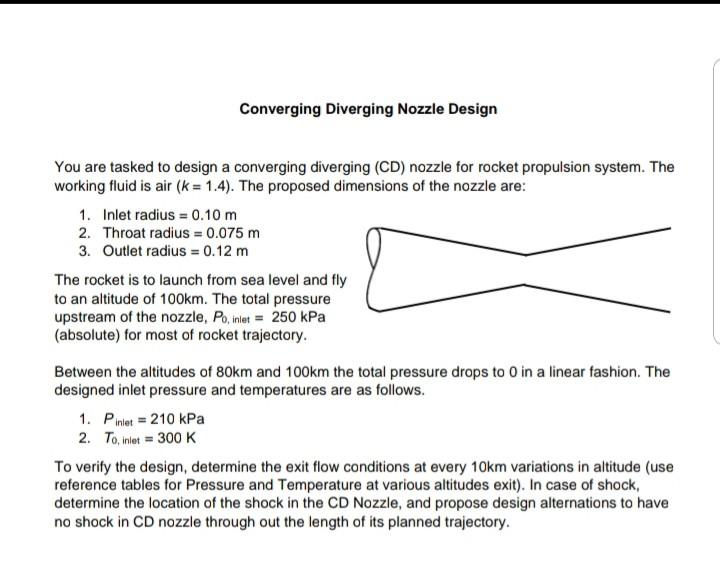# Question Converging Diverging Nozzle Design You are tasked to design a converging diverging (CD) nozzle for rocket propulsion system. The working fluid is air (k = 1.4). The proposed dimensions of the nozzle are: 1. Inlet radius = 0.10 m 2. Throat radius = 0.075 m 3. Outlet radius = 0.12 m The rocket is to launch from sea level and fly to an altitude of 100km. The total pressure upstream of the nozzle, Po, inlet = 250 kPa (absolute) for most of rocket trajectory. Between the altitudes of 80km and 100km the total pressure drops to 0 in a linear fashion. The designed inlet pressure and temperatures are as follows. 1. Piniet = 210 kPa 2. To, inlet = 300 K To verify the design, determine the exit flow conditions at every 10km variations in altitude (use reference tables for Pressure and Temperature at various altitudes exit). In case of shock, determine the location of the shock in the CD Nozzle, and propose design alternations to have no shock in CD nozzle through out the length of its planned trajectory.NPGZSH The Asker · Mechanical EngineeringTranscribed Image Text: Converging Diverging Nozzle Design You are tasked to design a converging diverging (CD) nozzle for rocket propulsion system. The working fluid is air (k = 1.4). The proposed dimensions of the nozzle are: 1. Inlet radius = 0.10 m 2. Throat radius = 0.075 m 3. Outlet radius = 0.12 m The rocket is to launch from sea level and fly to an altitude of 100km. The total pressure upstream of the nozzle, Po, inlet = 250 kPa (absolute) for most of rocket trajectory. Between the altitudes of 80km and 100km the total pressure drops to 0 in a linear fashion. The designed inlet pressure and temperatures are as follows. 1. Piniet = 210 kPa 2. To, inlet = 300 K To verify the design, determine the exit flow conditions at every 10km variations in altitude (use reference tables for Pressure and Temperature at various altitudes exit). In case of shock, determine the location of the shock in the CD Nozzle, and propose design alternations to have no shock in CD nozzle through out the length of its planned trajectory.
More
Transcribed Image Text: Converging Diverging Nozzle Design You are tasked to design a converging diverging (CD) nozzle for rocket propulsion system. The working fluid is air (k = 1.4). The proposed dimensions of the nozzle are: 1. Inlet radius = 0.10 m 2. Throat radius = 0.075 m 3. Outlet radius = 0.12 m The rocket is to launch from sea level and fly to an altitude of 100km. The total pressure upstream of the nozzle, Po, inlet = 250 kPa (absolute) for most of rocket trajectory. Between the altitudes of 80km and 100km the total pressure drops to 0 in a linear fashion. The designed inlet pressure and temperatures are as follows. 1. Piniet = 210 kPa 2. To, inlet = 300 K To verify the design, determine the exit flow conditions at every 10km variations in altitude (use reference tables for Pressure and Temperature at various altitudes exit). In case of shock, determine the location of the shock in the CD Nozzle, and propose design alternations to have no shock in CD nozzle through out the length of its planned trajectory.